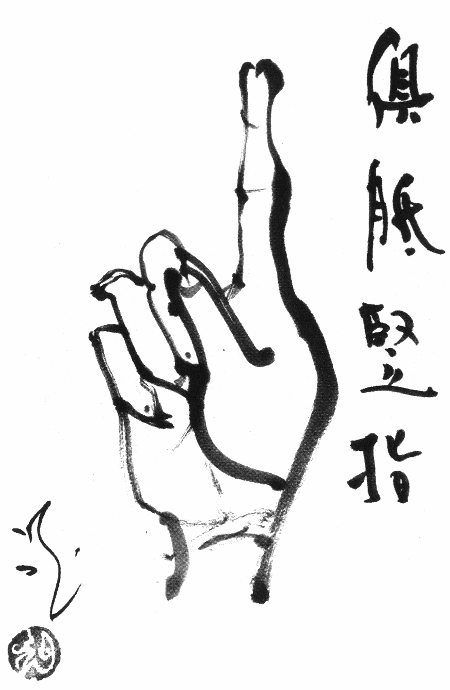## Ψ.1.Natural repetition

### # 1.3.Capacity of countingWhenever Master Judi was questioned, he would just raise a finger. Later a servant boy would also raise a finger when outsiders asked him what the master taught.
When Judi heard of this, he cut off the boy's finger with a knife.
The boy ran out screaming in pain, but Judi called him back. When the boy turned his head, Judi raised a finger. Suddenly the boy attained enlightenment.
When Judi was about to die, he said to a group, "I attained my teacher Tianlong's one-finger Zen, and have used it all my life without exhausting it." So saying, he passed away.

Wumenguan 3.

#### §1.3.1.Fingers forever

Our choice to count fingers `1..` for the final representation of Big numbers is not so primitive as you might think. For practical Big purposes the capacity of a series of `1..` is comparable to decimal numbers. Whether or not scientific exponential notation m.nEp `=` m.n×10^p is applied doesn't even matter.
In this section we'll prove our point, by considering an example number, moderately large in size.

10^^10 = 10^... {10#10}
= 1E1E1E1E1E1E1E1E1E10  with 9 exponents E

In totally reduced form we print this number out with that many units `1`. Can you do better in any radix `10`?
A series of `n` ones can be noted down in `log(n)` digits, concisely so it seems. But when numbers are large enough, as is `n = 10^^10` in decimal base, even scientific notation doesn't offer adequate storage capacity.

log(10^^10) = log(10^10^^(10-1)) = 10^^(10-1)
10^^9 decimal digits, or 10^^8 after exponent E

It's a waste to try to print out Big numbers, either as series of `1..` or as decimal numbers. If all the land on every planet in the universe was thick with thin paper production forests, you could write at most 10^50 characters – go figure how many universes you need!
Such an approach bears no fruit, because it is in essence division and Bigness stays approximately the same when divided. Big numbers can only be physically represented in a mathematical expression. The algorithm which justifies their existence isn't really meant to reduce expressions – not to series of units `1` and not to digits in any radix.

The principle of frugality then tells us not to waste characters to support an inefficient number base. Our algorithms can do without even a single `0` and our unit machine functions with compelling logic.
We can use two natural numbers to represent a fraction n/m or replace a decimal point n.m
The resolution of finger counting is the same as in a radix system – all natural numbers are uniquely covered.

##### Word of appreciation

Anything can be counted by repeating the unit one. Once a new world is discovered from the outside, the inside can be referred to with a single finger. (Jiun says, "Discover it from the inside!") Mathematically this is very Big, but if the finger points at itself, it is just the initial number one again, a humble finger, humbler than us maybe. (Sits in silence.)
I count myself lucky (:o) to have a finger that contains the universe. I want to show it more often! (Sounds the bell.)

#### §1.3.2.Out of sight

Where lies the boundary of our physical world view? And where do binary power towers `2^^n` `= 2^...` {2#n} effectively stop increasing when squared and stop decreasing by `√` square roots? We'll show you below how to scale down to division with Big numbers. For a more humane limit, click here!

2^^6^2 = 2^(2^^5*2) (square)
= 2^2^(2^^4+1) = 2^2^65537
= 2^2^2^(2^^3+lg(1+1/2^^4)) ~ 2^2^2^16.000022
~ 2^2^2^2^2^(2+2^-20.4) ~ 2^2.000021^2^^4
= 4^2^^5 ~ (2+2^-22.4)^^6

√(2^^7) = 2^2^(2^^5-1) (square root)
~ 2^2^2^(2^^4+lg(1-1/2^^5))
~ 2^2^2^(65536-7E-19729)
= √2^2^^6 ~ 2^^7

10^^3/2 ~ 10^(10^^2-0.301) (half)
~ 1E1E9.999999999987 ~ (10-7E-10)^10^^2
~ (10-9E-12)^^3

The function lg above refers to logarithms of `2` so that lg(a) `= log(a)/log(2)`
With respect to a number created by tetration `a^^b` squaring proves equally insignificant as adding `1`, in that both operations lack the capacity to set most numbers `2^^b` and `2^^b1` visually apart.

A number system with a resolution where squares have meaning is the bottom line in the definition of scientific measures. Apparently our quantity modelling comedy capers capabilities lose eye contact at the 3d storey (2nd exponent) and the basic qualities of physical information drop out of sight when a power tower reaches its `7th storey`.

From the perspective of a recursion theorist a mathematical expression is input, formulated by using two or more character-types. A reduction scheme or program simplifies this expression in several recursive steps, which typically require extra bracket character(s). But the projected output will be a number of repeats of just the unit one `1..`
Where computability matters, to squander our signs on luxury digits other than `1` would be un-mathematical.